# 3317) Let T be a linear transformation from R° to R, andu and v be orthogonal vectors. Which of thefollowing pairs of vectors must be orthogonal?a) Tu and ub) Tv and vTu and Tvc)d)ru and sv (where r and s are real numbers)e) u+v and u-v

Question
1 views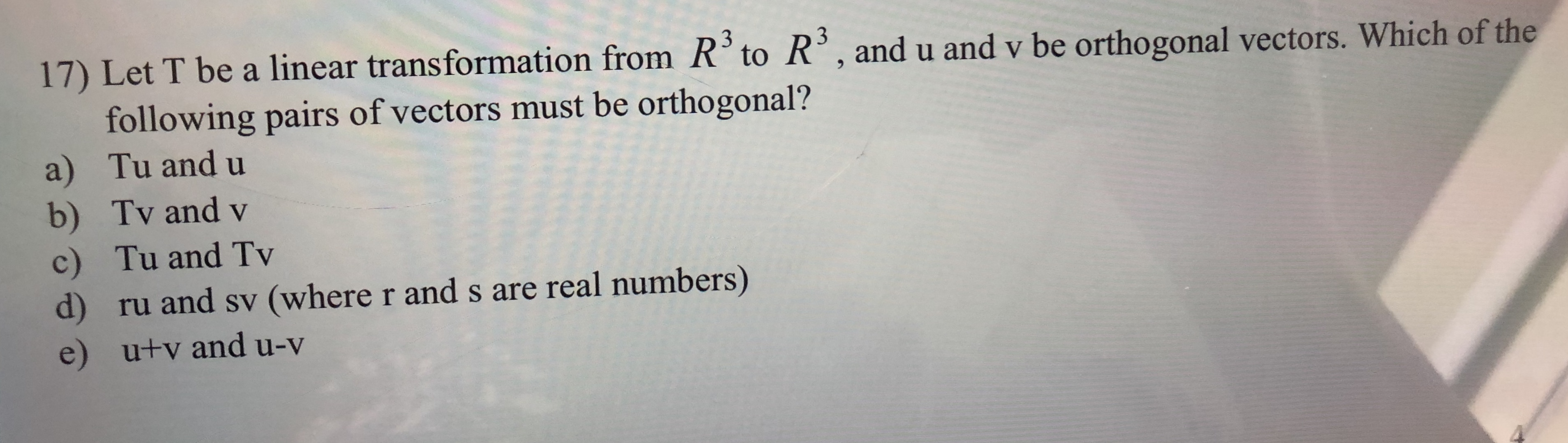help_outlineImage Transcriptionclose3 3 17) Let T be a linear transformation from R° to R, andu and v be orthogonal vectors. Which of the following pairs of vectors must be orthogonal? a) Tu and u b) Tv and v Tu and Tv c) d) ru and sv (where r and s are real numbers) e) u+v and u-v fullscreen
check_circle

Step 1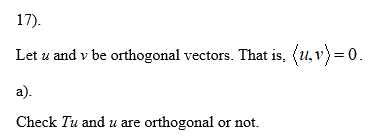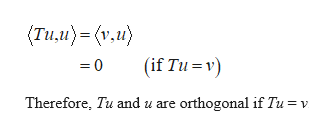help_outlineImage Transcriptionclose(Tим)- (v.и} (if Tu v) =0 Therefore, Tu and u are orthogonal if Tu = v fullscreen
Step 2

b)

Check Tv and v are orthogonal or not.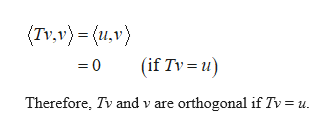help_outlineImage Transcriptionclose(Tv.v)= (u,v) (if Tv u) =0 Therefore, Tv and v are orthogonal if Tv = u fullscreen
Step 3

c)

Check Tu and Tv are orthogon...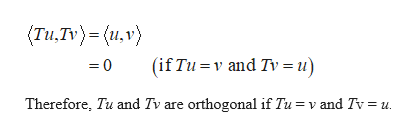help_outlineImage Transcriptionclose(Tи,Tv)-(и,v) (if Tu v and Tv = u) =0 Therefore, Tu and Tv are orthogonal if Tu = v and Tv = u fullscreen

### Want to see the full answer?

See Solution

#### Want to see this answer and more?

Solutions are written by subject experts who are available 24/7. Questions are typically answered within 1 hour.*

See Solution
*Response times may vary by subject and question.
Tagged in